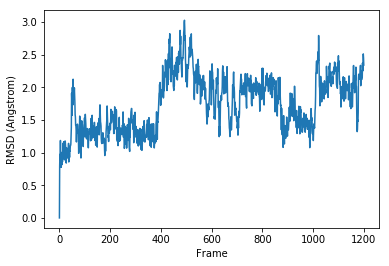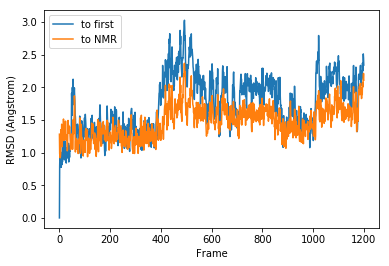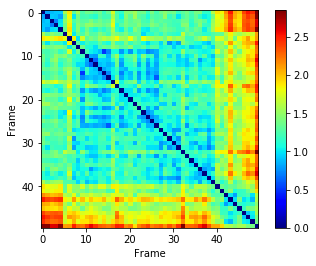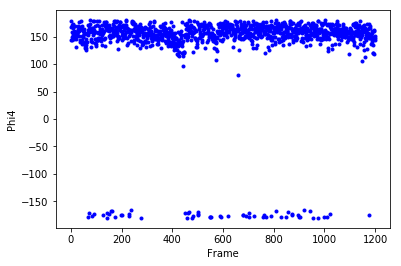AmberTools21 Amber20 Manuals Tutorials Force Fields Contacts HistoryUseful links: Amber Home Download Amber Installation Amber Citations GPU Support Updates Mailing Lists For Educators File Formats

# Interactive data analysis with pytraj/cpptraj and Jupyter notebook ¶

## What is Jupyter notebook?¶

Direct introduction and figure from Jupyter notebook website:

"The Jupyter Notebook is a web application that allows you to create and share documents that contain live code, equations, visualizations and explanatory text. Uses include: data cleaning and transformation, numerical simulation, statistical modeling, machine learning and much more."

<img src="images/jupyterpreview.png", width=500>

## What is pytraj?¶

pytraj is a Python package binding to the popular cpptraj program. pytraj is written do extend the flexibility of cpptraj and to expose cpptraj's functionality to Python's ecosystem, such as numpy, pandas, matplotlib, ...

## What can you learn from this tutorial¶

• Use Jupyter notebook for interactive data exploration

• Use pytraj to perform basis analysis such loading files to memory and do computing RMSD

• Use matplotlib and pandas for analyzing output from pytraj

## Requirement¶

• AmberTools >= 16
• If you allow AMBER to install Python distribution from Miniconda, you have most needed packages (except pandas) for this tutorial.
• If not, you need to install (either using pip or conda)
• matplotlib
• jupyter notebook
• Install pandas:
amber.conda install pandas

• You know how to use Linux command line. If not, please check basic AMBER tutorial

All files used in this tutorial, including this notebook, is here: tutorial_files.zip

mkdir tutorial
cd tutorial
unzip ../tutorial_files.zip


## Start notebook¶

\$ amber.jupyter notebook
(or jupyter notebook if you did not allow AMBER to install Miniconda)

• You should expect to see a blank directory (or with a list of files)• Choose New --> Python 2 (or Python 3, depending on which version you're using)

• You should expect to see• How to follow this tutorial? Copy and paste each command in this tutorial to the notebook, hit "Shift-Enter" to run and to create new Cell. Check Tips in the end of this tutorial for useful commands.

• If you want to use the notebook remotely (run it in your remote cluster and view it in your desktop), please check remote notebook tutorial

• Note: This tutorial is written in the Jupyter notebook, so you can download and run it too.

In :
from __future__ import print_function
import warnings
warnings.filterwarnings("ignore", category=DeprecationWarning) # avoid warning message to make the notebook nicer

import pytraj as pt



## Get some basic information about the trajectory¶

In :
print(traj)

pytraj.Trajectory, 1201 frames:
Size: 0.000000 (GB)
<Topology: 220 atoms, 13 residues, 1 mols, non-PBC>



## Computing RMSD, using 1st frame as reference¶

In this case we are computing mass-weighted RMSD, using all non-hydrogen atoms in residues 1 to 13

In :
data_rmsd_first = pt.rmsd(traj, ref=0, mask=":1-13&!@H*")

print(data_rmsd_first)

[  2.30050392e-07   7.92393865e-01   9.72257525e-01 ...,   2.51047183e+00
2.44805673e+00   2.33894156e+00]


### Using matplotlib to plot¶

In :
%matplotlib inline

from matplotlib import pyplot as plt

plt.plot(data_rmsd_first)
plt.xlabel('Frame')
plt.ylabel('RMSD (Angstrom)')

Out:
<matplotlib.text.Text at 0x127834b90>In :
# we can reuse loaded topology from traj
print(ref)

pytraj.Trajectory, 1 frames:
Size: 0.000000 (GB)
<Topology: 220 atoms, 13 residues, 1 mols, non-PBC>



## Computing RMSD to a Reference¶

In :
data_rmsd_ref = pt.rmsd(traj, ref=ref, mask=":1-13&!@H*")
print(data_rmsd_ref)

[ 1.28171363  0.98184973  0.95976186 ...,  2.1723379   2.21177339
2.10760399]


## Plotting two RMSDs¶

We can plot rmsd to 1st frame (previously calculated) and rmsd to reference (NMR)

In :
plt.plot(data_rmsd_first, label='to first')
plt.plot(data_rmsd_ref, label='to NMR')
plt.xlabel('Frame')
plt.ylabel('RMSD (Angstrom)')
plt.legend()

Out:
<matplotlib.legend.Legend at 0x12ae74c90>## Computing pairwise RMSD for specific snapshots¶

In :
# compute pairwise RMSD for first 50 snapshots and skip every 10 frames
mat = pt.pairwise_rmsd(traj, mask=":1-13&!@H*", frame_indices=range(0, 500, 10))
print(mat)

[[ 0.          0.81742334  0.88945633 ...,  2.52540493  2.44746494
2.83389616]
[ 0.81742334  0.          0.92505556 ...,  2.42510176  2.32717562
2.82156968]
[ 0.88945633  0.92505556  0.         ...,  2.34702587  2.26490974
2.60315514]
...,
[ 2.52540493  2.42510176  2.34702587 ...,  0.          1.00938892
1.4123441 ]
[ 2.44746494  2.32717562  2.26490974 ...,  1.00938892  0.          1.39651227]
[ 2.83389616  2.82156968  2.60315514 ...,  1.4123441   1.39651227  0.        ]]

In :
plt.imshow(mat, cmap='jet')
plt.xlabel('Frame')
plt.ylabel('Frame')
plt.colorbar()

Out:
<matplotlib.colorbar.Colorbar at 0x12aec9790>## Computing dihedral angles¶

In this case, we are computing phi and psi angle for residues 2 to 12. We tell pytraj to convert raw data to pandas's DataFrame to better visualization in notebook

In :
phipsi = pt.multidihedral(traj, resrange='1-12', dihedral_types="phi psi", dtype='dataframe')


### Pretty display data¶

In this case, we only display a part of the data

In :
phipsi[['phi_4', 'psi_4', 'phi_5', 'psi_5']].head(5)

Out:
phi_4 psi_4 phi_5 psi_5
0 -75.439203 136.828950 -166.265268 142.963215
1 -83.644329 130.036725 -149.613451 140.469441
2 -97.466250 138.200851 -152.872485 122.812971
3 -90.913898 115.232213 -126.890970 132.339599
4 -94.040970 131.921428 -140.411407 151.705385

### Getting some basic info¶

In :
phipsi[['phi_4', 'psi_4', 'phi_5', 'psi_5']].describe()

Out:
phi_4 psi_4 phi_5 psi_5
count 1201.000000 1201.000000 1201.000000 1201.000000
mean -84.227620 131.166893 -143.707816 105.065698
std 16.317879 12.427970 13.340660 39.313901
min -147.577326 80.866604 -176.154622 -179.166655
25% -94.353636 123.182218 -152.771024 71.362192
50% -82.585343 131.887478 -145.219714 115.641876
75% -73.350432 140.019820 -136.516230 134.998512
max -37.436428 166.331044 -64.705252 176.879019

### Plotting dihedral¶

In this case, we are plotting Psi dihedral for residue 4 (TRP)

In :
plt.plot(phipsi['psi_8'], '-bo', markersize=3, linewidth=0)
plt.xlabel('Frame')
plt.ylabel('Phi4')

Out:
<matplotlib.text.Text at 0x12c783d90># Get some information about the structure¶

In :
top = traj.top

for residue in top.residues:
# Note: In python, 0-based index is used.
# For example: SER0 should be first residue
print(residue)

<SER0, 13 atoms>
<TRP1, 24 atoms>
<THR2, 14 atoms>
<TRP3, 24 atoms>
<GLU4, 15 atoms>
<ASN5, 14 atoms>
<GLY6, 7 atoms>
<LYS7, 22 atoms>
<TRP8, 24 atoms>
<THR9, 14 atoms>
<TRP10, 24 atoms>
<LYS11, 22 atoms>
<NHE12, 3 atoms>

In :
list(traj.top.atoms)[:10]

Out:
[Atom(name=N, type=N3, atomic_number=7, index=0, resid=0),
Atom(name=H1, type=H, atomic_number=1, index=1, resid=0),
Atom(name=H2, type=H, atomic_number=1, index=2, resid=0),
Atom(name=H3, type=H, atomic_number=1, index=3, resid=0),
Atom(name=CA, type=CX, atomic_number=6, index=4, resid=0),
Atom(name=HA, type=HP, atomic_number=1, index=5, resid=0),
Atom(name=CB, type=CT, atomic_number=6, index=6, resid=0),
Atom(name=HB2, type=H1, atomic_number=1, index=7, resid=0),
Atom(name=HB3, type=H1, atomic_number=1, index=8, resid=0),
Atom(name=OG, type=OH, atomic_number=8, index=9, resid=0)]

## Tips¶

• Run bash command: Use !
In :
! echo Hello

Hello

• How to run this notebook?

• Run all commands: Cell -> Run All
• Run each Cell: Ctrl-Enter
If you would like to learn more about pytraj, please see unofficial AMBER tutorials for pytraj in pytraj website